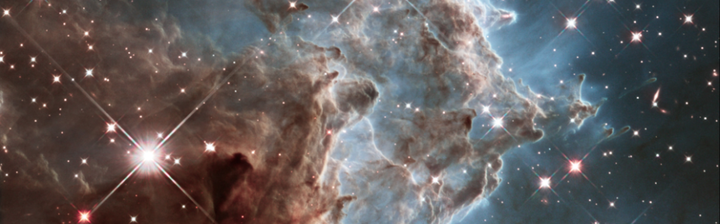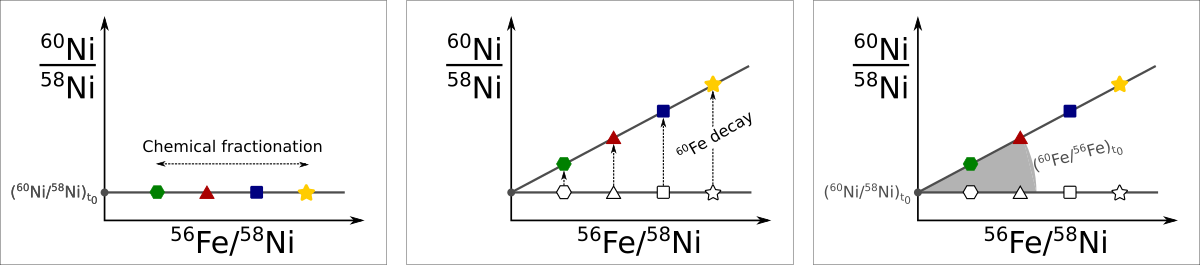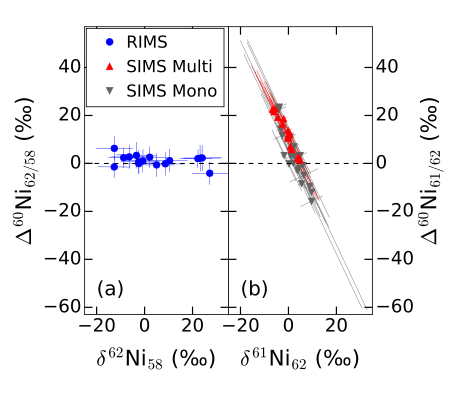# The Solar Nebula## The composition of the solar nebula

The solar system formed around 4.5 billion years ago, which is around 9 billion years after the formation of the Milky Way itself. This means that the average solar composition that the solar nebula started with represents one datapoint in terms of galactic chemical evolution.

The solar composition can be measured excellently by observing the sun as well as by analyzing primitive meteorites. Except for volatile species, e.g., hydrogen and the noble gases, the composition of these two sample sets agree very well with each other. However, there are some anomalies that can be found in meteorites, which are very indicative of the exact circumstances under which the solar system formed.

Some of the anomalies that can be found in primitive objects from the solar system are enhancement in certain isotopes due to the in situ radioactive decay of short-lived radionuclides (SLRs). Calcium aluminum-rich refractory inclusions (CAIs), which are fluffy looking phases in primitive meteorites, are some of the very first material that condensed in the solar nebula and are the ideal samples to look for such anomalies.

### Determining the abundance of SLRs in the solar nebula

If for example the short-lived 60Fe was incorporated into a solid that condensed early on in the solar system we would be able to measure it today as an enhancement of 60Ni since the original 60Fe, which has a half-life of 2.6 Ma, would have all decayed to its stable daughter by now. The figure below shows how the presence of SLRs can be detected in a given sample.The figure on left shows the scenario at formation. Different minerals condense with different amounts of iron to nickel elemental compositions. The figure in the middle shows what happens with these different phases once they are solidified. The y-axis here always shows the 60Ni/58Ni ratio. The more iron a phase condensed with the more 60Fe it will have incorporated as well. Thus, once all the 60Fe has decayed to 60Ni the phases will end up with different 60Ni/58Ni ratios. The figure on the right shows the scenario today. By measuring different phases for their Fe/Ni elemental ratio (shwown on the x-axis) and their nickel isotopic composition (y-axis), the original value of the 60Fe/56Fe at the time of condensation can be determined by fitting a line to these measurements. In above example this line will have the form:

$$\frac{^{60}\rm{Ni}}{^{58}\rm{Ni}} = \left(\frac{^{60}\rm{Fe}}{^{56}\rm{Fe}}\right)_ 0 \times \frac{^{56}\rm{Fe}}{^{58}\rm{Ni}} + \left(\frac{^{60}\rm{Ni}}{^{58}\rm{Ni}}\right)_ 0$$

Here, the isotope ratios with subscript 0 represent the initial composition of the isotope ratio when the phase condensed. The original 60Fe/56Fe ratio is thus nothing else than the slope of the regression.

Assuming that an SLR was distributed homogeneously in the early solar system allows to also date phases relative to each other. For example, if one phase shows half the initial 60Fe/56Fe than another phase we can say that the phase with less initial 60Fe condensed one 60Fe half life (2.6 Ma) after the first one.

### The presence of 26Al and its effects

One of the most prominent SLRs that has been shown to be present in the solar nebula is 26Al (half life 717 ka). Many studies have shown that 26Al was distributed homogeneously in the solar nebula and that its initial abundance when CAIs formed was $$^{26}\rm{Al}/^{27}\rm{Al} = 5\times10^{-5}$$. This has important implications because it allows us to date different phases in the early solar system relative to each other. Furhtermore, when 26Al decays to 26Mg it releases 4 MeV of energy. This is a significant amount and has been shown to be able to heat an asteroid parent body of a given size (and thus of a given 26Al content) significally. In fact, 26Al has been invoked as being a crucial heat source that started the differentiation of early planetesimals.

The presence of 26Al in the solar system raises the quesion on where it came from. Since its half-life is only 717 ka it had to be produced just prior to solar system formation. The key to decipher where it came from comes from studying other SLRs that were also present in the solar nebula.

### The curious case of 60Fe in the solar nebula

One nuclide that can help us solve the question on where the 26Al in the early solar system came from is 60Fe. If 60Fe is also present in the solar nebula it was likely produced and injected along with 26Al. A large amount of 60Fe in the early solar nebula, by which we mean a value of $$^{60}\rm{Fe}/^{56}\rm{Fe} > 5\times10^{-7}$$ would make it likely that a supernova that exploded just before the solar system produced and injected the two nucldies. A low value of $$^{60}\rm{Fe}/^{56}\rm{Fe} \sim 10^{-8}$$ would however indicate that no such supernova took place and that the 60Fe does not need to be produced but is rather the galactic background value.

This discussion might seem very fabricated, however, for years and years the community has argued over the amount of 60Fe present in the solar nebula and the mentioned values are not just randomly picked. In situ studies of chondrules, objects that formed in the solar system about 2 Ma after CAIs, by secondary ion mass spectrometry (SIMS) showed high 60Fe values and thus pointed towards a supernova source. Bulk studies of many meteorites on the other hand consistently measured low 60Fe values. Until recently, the in situ measurements could not be tested with other techniques than SIMS.

In a recent publication we re-analyzed one chondrule with resonance ionization mass spectrometry (RIMS). This sample has previously only been studied with SIMS. RIMS has the advantage over SIMS that all nickel isotopes can be measured without interferences. SIMS however can only anlyze 60Ni, 61Ni, and 62Ni. In order to determine the excess in 60Ni the measurements needs to be normalized to another isotope (see isochron figure above). Furthermore, mass dependent fractionation also has to be corrected for, thus measuring a third isotope is required.The above figure shows the new results obtained by RIMS (left, Trappitsch et al., 2018) versus a re-evaluation of the old results by SIMS (right, Telus et al., 2018). To evaluate the SIMS measurements requires doing the described corrections with the low abundance isotope 61Ni. This results in extreme correlations of the uncertainties, which, if not adequately accounted for, can seem like excesses in 60Ni due to in situ decay of 60Fe. With RIMS however this problem does not exist. The figure on the left clearly shows that the errors are uncorrelated thanks to the ability to measure 58Ni.

Our recent re-evaluation has brought up issues with the data processing that has been done in the determination of the initial 60Fe abundance. It remains to be seen if this is the case for all samples, however, preliminary evaluations of other SIMS measurements show that the same problem occurs in the rest of the dataset. A supernova thus cannot have injected the 26Al and another source, e.g., a Wolf-Rayet star, is required to explain its high value in the solar nebula.